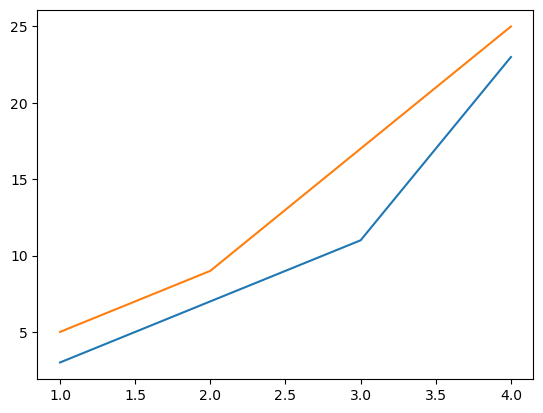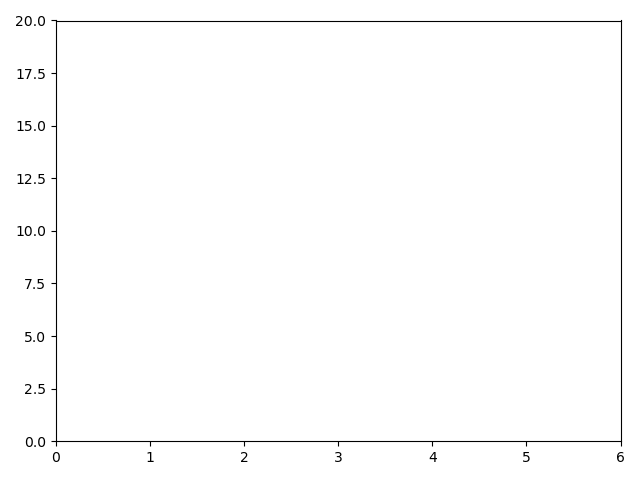# # Matplotlib Figures, Plots, and Axes

Quick Definition

A figure contains one or more groups of axes.

Each Axes, which can be 2-dimensional or 3-dimensional, contains zero or more plots.

A plot is the thing we normally call "graph" or "chart".

## # Figure

The `Figure` is the top level container. Inside a figure we can include one or more `Axes` classes, each of which can have one or more plots.

The `Figure` is what contains everything else, and it is also what we show when we want to display the graph to the screen:

``````import matplotlib.pyplot as plt

plt.figure()
plt.show()
``````

Here we've used the `pyplot` library to create a new `Figure`. When we do this, `pyplot` automatically "selects" the figure, and it becomes the active figure. Every other function in the `pyplot` library will act on that figure until another one is selected.

But note if you run that code, you'll see no output.

The figure is empty, so matplotlib doesn't display a window.

We must first add at least a set of axes to the figure.

## # Axes

The `Axes`, which is a matplotlib class, is where plots are drawn. You can have multiple plots drawn on a single pair of axes, as below:Or you can also have multiple `Axes` in one figure, as below:Let's start off by adding a set of 2-dimensional axes to our figure:

``````import matplotlib.pyplot as plt

plt.figure()
plt.axis([0, 6, 0, 20])
plt.show()
``````Here we've used `plt.axis()` to define the values in our x and y axes. The x axis goes from `0` to `6`, and the y axis goes from `0` to `20`.

Note that `plt.axis()` acts on the currently active figure, which is the one that got created when we called `plt.figure()`.

At the moment there's nothing drawn in the canvas though, so let's do that!

## # Plot

Plots can be drawn on a set of axes, and each plot needs to specify data points for the axes that it is drawn on.

For example, if we have 2-dimensional axes, then for each data point we need to specify its x and y coordinates.

``````import matplotlib.pyplot as plt

plt.figure()
plt.axis([0, 6, 0, 20])
plt.plot([1, 2, 3, 4], [3, 5, 9, 25])
plt.show()
``````

Here, we use `.plot()` to draw 4 points in our axes. The points drawn are:

• `(1, 3)`
• `(2, 5)`
• `(3, 9)`
• `(4, 25)`

Note that the y axis only goes up to 20 though, so therefore this plot will be clipped at 20 in the y axis, and it won't be shown in its entirety:If we don't specify the axes dimensions ourselves, `.plot()` will do it for us based on the provided data:

``````import matplotlib.pyplot as plt

plt.figure()
plt.plot([1, 2, 3, 4], [3, 5, 9, 25])
plt.show()
``````Note the y axis here goes to 25, since that's the maximum value in our data set.